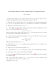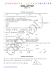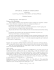# Math 3230 (Roby) Practice Midterm Fall 2014 Solutions

## Transcription

Math 3230 (Roby) Practice Midterm Fall 2014 Solutions
```Math 3230 (Roby)
Practice Midterm Solutions
Fall 2014
1. Decide whether each statement below is Always, Sometimes, or Never True. Justify
(a) If |a2 | = |b2 |, then |a| = |b|. Sometimes. In D4 it’s true when a = H and b = V
and false when a = H and b = R0 .
(b) The set {1, 2, . . . , n − 1} is a group under multiplication modulo n. Sometimes.
True when n is prime. False when n = 6, since 2 · 3 = 0, or since 2 and 3 have no
multiplicative inverse.
(c) Let |G| = p, where p is prime. Then G is cyclic.
Always true, since any
non-identity element x ∈ G must have order p by Lagrange’s theorem.
(d) Every cyclic group of order n is isomorphic to Zn .
generator g of the cylic group to 1 ∈ Zn .
Always true. Map the
2. For each pair of groups below, decide whether they are isomorphic or not and prove
(a) Z4 and U (8) Not isomorphic since Z4 has an element of order 4, while each
element of U (8) has order 1 or 2.
(b) A4 and D6 . Not isomorphic, since in A4 every element has order 4,3,2, or 1,
while R60 ∈ D6 has order 6.
(c) 2Z and 3Z. Isomorphic via the map t 7→ 23 t.
(d) Aut(Z18 ) and Z12 . Should have been Z6 ! Aut(Z18 ) ∼
= U (18) = {1, 5, 7, 11, 13, 17} =
∼
h5i = Z6 .
3. Find all β ∈ S7 such that β 4 = (2134657). Find all γ ∈ S9 such that γ 4 = (2134657).
Since β 28 = (β 4 )7 = (1), we know β divides 28. Since β 4 6= (1), we know β 6= 1, 2, or
4. But for β to have order 14 or 28, it would need (at a minimum) to have cycles of
length 7 and 2 or 7 and 4. Hence, |β| = 7. So now β = β 8 = (β 4 )2 = (2367145). For
γ ∈ S9 , we can either get (2367145)(8)(9) (of order 7) or (2367145)(89) (of order 14).
4. Let G be a group and g ∈ G. If z ∈ Z(G), show that the inner automorphism induced
by g is the same as the inner automorphism induced by zg (i.e., the mappings φg and
φzg are equal). Since φg (y) = gyg −1 and φzg (y) = (zg)y(zg)−1 = zgyg −1 z −1 = gyg −1
since z ∈ Z(G). Hence, φzg = φg .
5. Suppose d is a positive divisor of 2n. How many elements of order d are there in Dn ?
(Hint: Consider the case d = 2 separately.) Dn has n reflections, each of order 2. Dn
also has n rotations, which form a cyclic subgroup of order n. By Thm. 4.4, there are
ϕ(d) rotations of order d. If n is odd, Dn has no rotations of order 2; if n is even, then
there is ϕ(2) = 1 rotation of order 2 (namely R180 ). So Dn has n + 1 elements of order
2 when n is even, and n when n is odd. For any d > 1 which divides 2n, there are ϕ(d)
elements of order d.
6. How many
elements of order 3 does S6 have? How many of these are in A6 ? There
are 63 · 2 = 40 permutations of cycle type [3, 1, 1, 1] and 12 63 · 2 · 2 = 40 permutations
of type [3, 3], for a total of 80. All of them are in A6
7. Prove or Disprove & Salvage (for extra credit!) if possible.
(a) If |G| = n and d | n, then G has a subgroup of order d. False. A4 has order 12
but no subgroups of order 6. (See text Example 7.5.) Salvage: True if G is cyclic.
(b) If α is an k-cycle and β is an `-cycle in Sn , then |αβ| = lcm(k, `). False: Let
α = (12) and β = (23). Then αβ = (123) has order 3 6= lcm(2, 2). Salvage: True
when cycles are disjoint.
(c) For any elements x and a in a group, |xax−1 | = |a|. A simple computation
shows that (xax−1 )k = xak x−1 = e ⇐⇒ xak = ex = xe ⇐⇒ ak = e (left
cancellation). Hence, their orders must be the same.
8. Suppose that H ≤ S4 and that H contains (12) and (234). Prove that H = S4 . By
closure H must contain (1234) = (12)(234) of order 4. Since H has elements of order
3 and 4, |H| ≥ 12 by Lagrange; but this isn’t enough. Notice that if we conjugate (12)
by (234), then we get (234)(12)(243) = (13); similarly, if we conjugate (12) by (234)−1 ,
we get (14). (A picture might help you understand the intuition behind this.) But
now we can get any transposition, since (1j)(1k)(1j) = (jk), which allows us to write
any permutation in S4 . (Can you generalize this argument to Sn ?)
9. Prove that a group of order 63 must have an element of order 3. Let a 6= e in G.
By Lagrange, |a| = 3, 7, 9, 21, or 63. If |a| = 3k, then |ak | = 3, and we’re done.
Otherwise, all non-identity elements of G have order 7, which is impossible, since 62 is
not a multiple of 6 = ϕ(7).
10. Go back over your old homework and quizzes to review and make sure you understand
any problem on which you lost points. Check!
11. Here are some specific tasks I expect you to be able to perform with demonstrated
understanding. In all of the following, G denotes a group.
(a) Decide whether a given set and operation forms a group or not.
(b) Given the Cayley (group operation) table for a group G, perform computations
in G and determine whether certain properties hold in G.
(c) Be able to prove basic properties of groups directly from the axioms.
(d) Decide whether a given subset of a group is actually a subgroup.
(e) Perform computations in and know basic properties of specific examples of groups
including: Zn , U (n), Sn , Dn , GL(2, F), SL(2, F), Z, Q+ , R∗ , C∗ , Rn .
(f) Disinguish between properties that only hold for finite groups as opposed to infinite ones, and only for cyclic groups.
(g) Know and be able to use the Fundamental Theorem of Cyclic Groups and other
basic facts about cyclic groups, e.g., how to find the number of elements of order
d in a cyclic group.
(h) Understand how to decompose a permutation into disjoint cycles and as a product
of transpositions and how to multiply permutations given as products of cycles.
Determine easily whether a permutation is odd or even.
(i) Know the statement and proof of Cayley’s Theorem: every finite group is a subgroup of SN for some N ∈ N.
(j) Understand what the order of an group and the order of an element are, and
how these are related to one another in a group. Be able to compute the order of
elements in various groups.
(k) Know the definitions of the center of a group, Z(G), and the centralizer of a
group element, C(a).
(l) Know the basic properties of isomorphisms of groups. Understand that the set
of isomorphisms of a group is itself a group, namely Aut(G), which contains the
subgroup Inn(G).
(m) Prove that two groups are isomorphic (by giving an explicit map and checking
that it’s an isomorphism) or that they are not isomorphic (by giving examples of
a property that only one of the two groups has).
(n) For H ≤ G, be able to define cosets of H in G and prove their basic properties,
particularly that they partition G into disjoint subsets.
(o) Know the statement of Lagrange’s Theorem, how to prove it, and some of its
applications.
(p) Know the Orbit-Stabilizer Theorem for a finite group acting on a set and be able
to apply it.
(q) Understand the theory of the course well enough to distinguish true statements
from false ones, giving proofs or counterexamples as appropriate.
```

### Pure Mathematics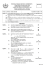### Sample Problems = +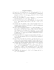### Sample Problems Math 334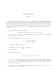### Document 6598654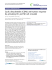### Solution to Sample Exam 1. Math 113 Summer 2014.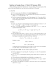### Groups Example Sheet 4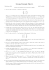### Sample Final Exam Questions (Mandatory Part)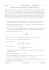### NSB 125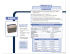### Charles E. Murry, MD, PhD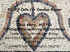### IT`S TIME TO PROVE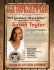### examples sheet - People